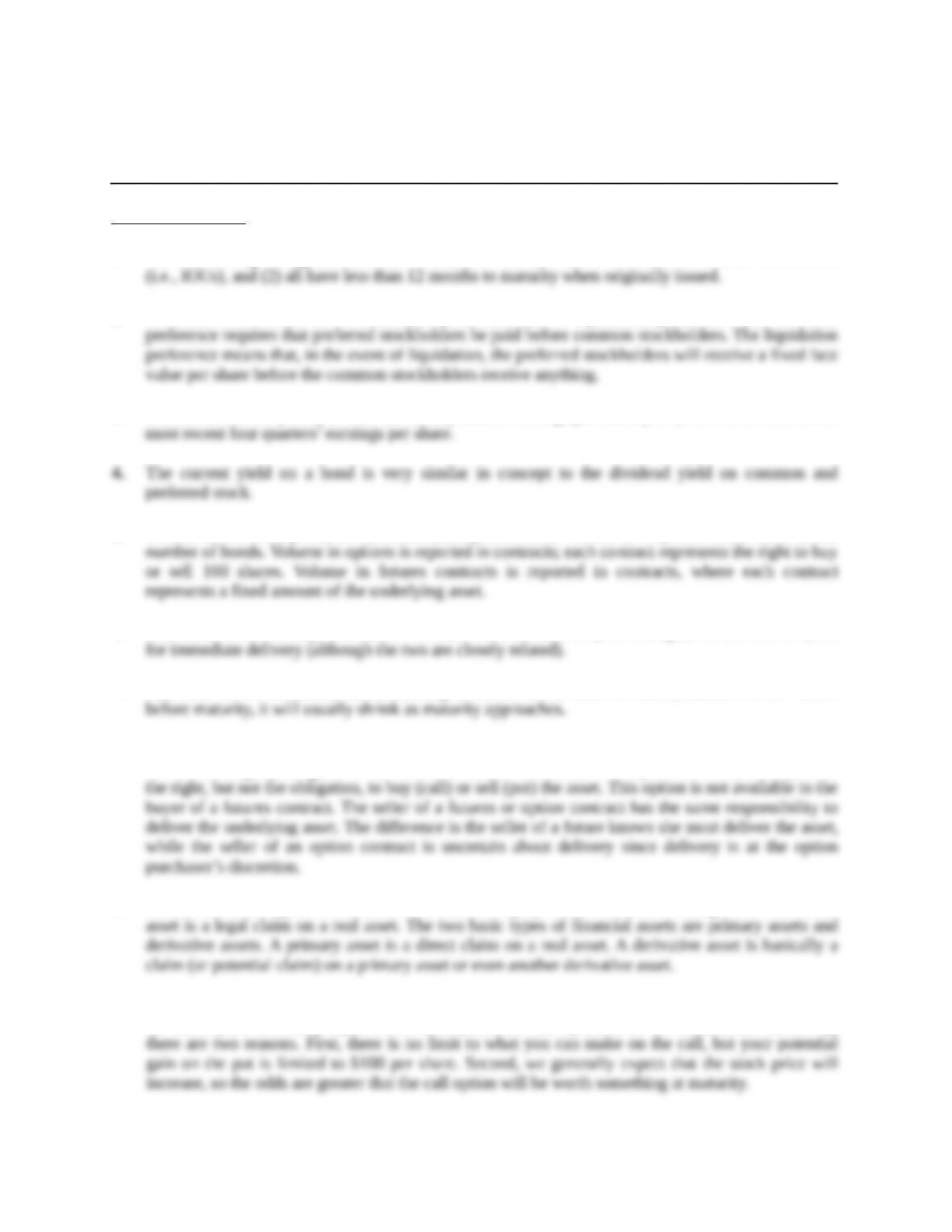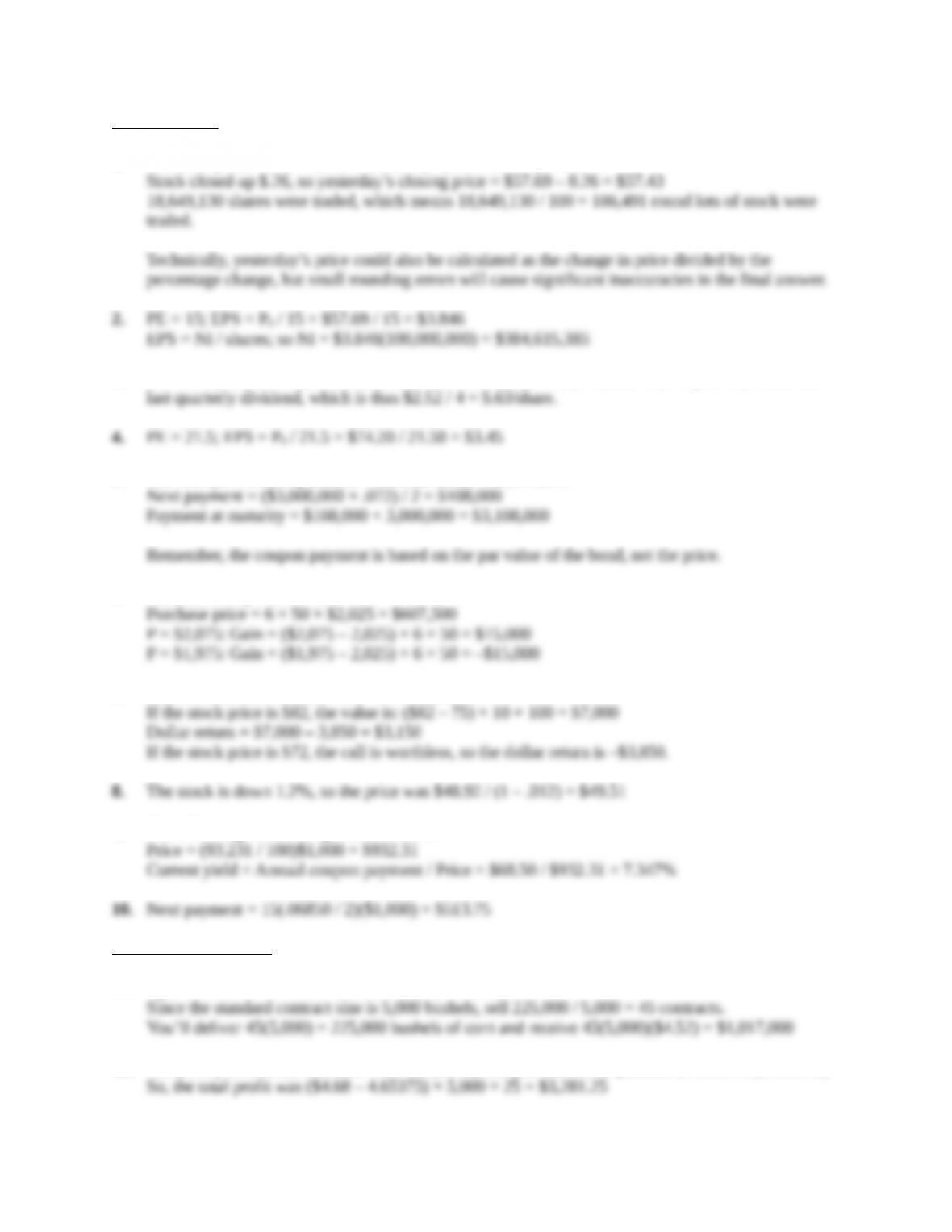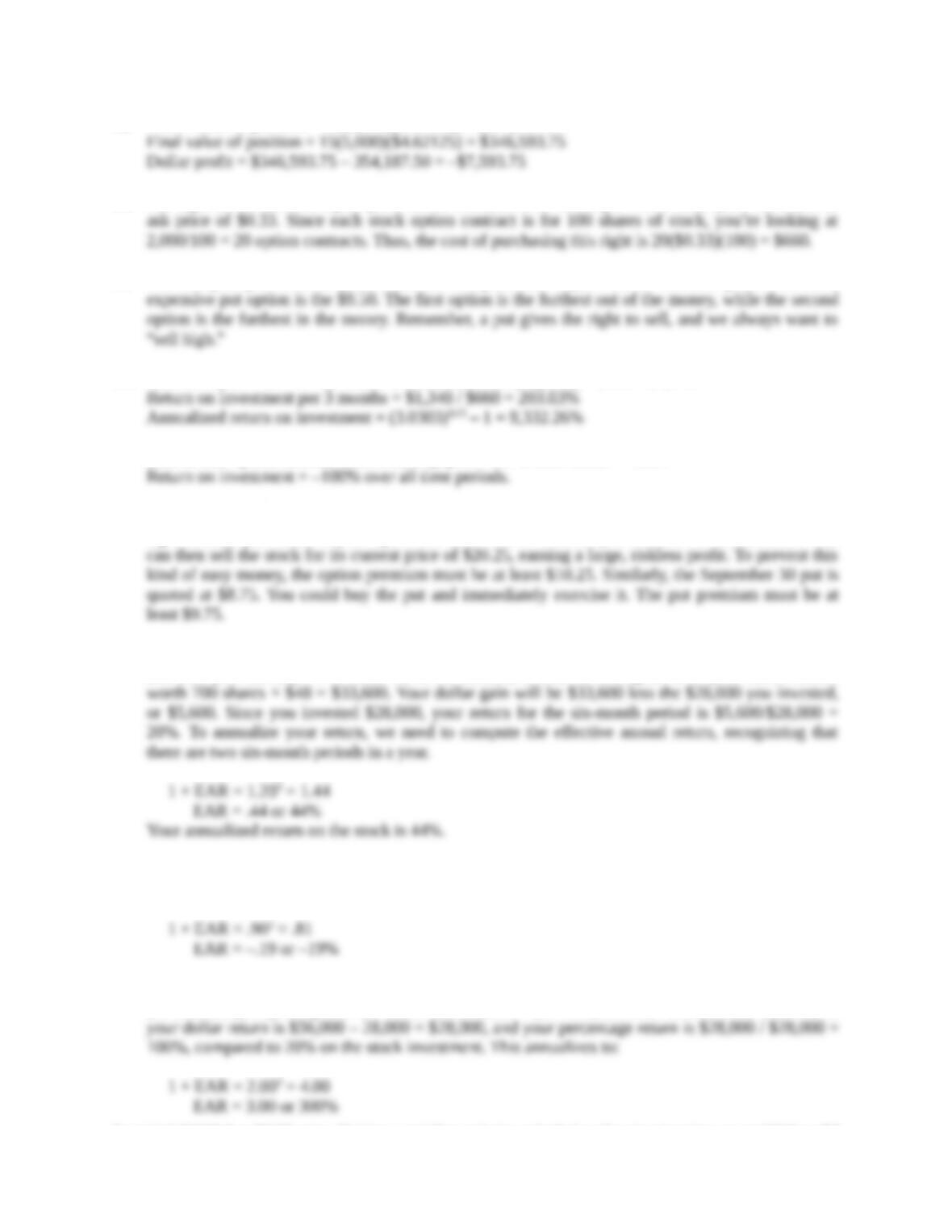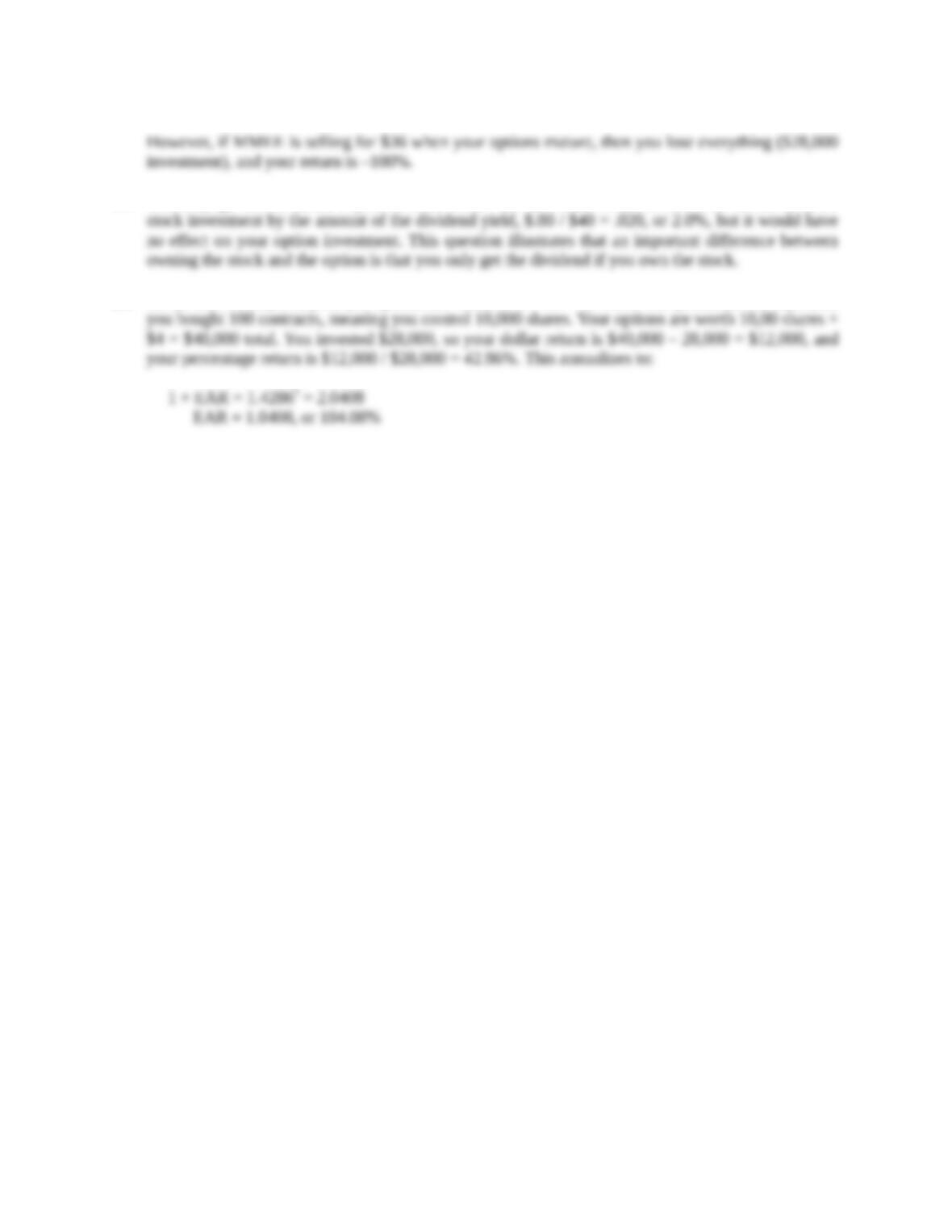Type
Solution Manual
Book Title
Fundamentals of Investments: Valuation and Management 8th Edition
ISBN 13
978-1259720697

### 978-1259720697 Chapter 3 Solution Manual

January 2, 2020Chapter 3
Overview of Security Types
Concept Questions
1. The two distinguishing characteristics are: (1) all money market instruments are debt instruments
2. Preferred stockholders have a dividend preference and a liquidation preference. The dividend
3. The PE ratio is the price per share divided by annual earnings per share (EPS). EPS is the sum of the
5. Volume in stocks is quoted in round lots (multiples of 100). Volume in corporate bonds is the actual
6. You make or lose money on a futures contract when the futures price changes, not the current price
7. Open interest is the number of outstanding contracts. Since most contract positions will be closed
8. A futures contact is a contract to buy or sell an asset at some point in the future. Both parties in the
contract are legally obligated to fulfill their side of the contract. In an option contract, the buyer has
9. A real asset is a tangible asset such as a land, buildings, precious metals, knowledge, etc. A financial
10. Initially, it might seem that the put and the call would have the same price, but this is not correct. If
the strike price is exactly equal to the stock price, the call option must be worth more. Intuitively,
Education.Core Questions
1. Dividend yield = .013 = \$.75 / P0 thus P0 = \$.75 / .013 = \$57.69
3. Dividend yield is 3.4%, so annualized dividend is .034(\$74.20) = \$2.52. This is just four times the
5. The total par value of purchase = 3,000(\$1,000) = \$3,000,000
6. Contract to buy = 300 / 50 = 6
7. Cost of contracts = \$3.85 × 10 × 100 = \$3,850
9. The YTM is given in the quote as 7.482%.
Intermediate Questions
11. Open interest in the March contract is 597,913 contracts.
12. The price you sold the contracts was 468 (\$4.68) and you closed the position at 465.375 (\$4.65375).
Education.13. Initial value of position = 15(5,000)(\$4.7225) = \$354,187.50
14. The right to sell shares is a put option on the stock; the November put with a strike price of \$9 has an
15. The cheapest put contract (that traded on this particular day) is the \$7 exercise price. The most
16. Case 1: Payoff = \$9 - 8 = \$1/share. Dollar return = \$1(20)(100) – \$660 = \$1,340
Case 2: The option finishes worthless, so payoff = \$0. Dollar return = –\$660
17. The very first call option listed has a strike price of 10 and a quoted premium of \$5.50. This can’t be
right because you could buy an option for \$5.50 and immediately exercise it for another \$10. You
18. If you buy the stock, your \$28,000 will purchase 700 shares, or 7 round lots. A call contract costs
\$400, so you can buy 70 of them. If, in six months, MMEE is selling for \$48, your stock will be
If MMEE is selling for \$36 per share, your loss on the stock investment is –10.00%, which
annualizes as follows:
At the \$48 price, your call options are worth \$48 40 = \$8 each, but now you control 7,000 shares
(70 contracts), so your options are worth 7,000 shares × \$8 = \$56,000 total. You invested \$28,000, so